Скачать презентацию LESSON 6 Position Displacement You have

e21b6fb6d5afc774604f491469416d96.ppt

• Количество слайдов: 8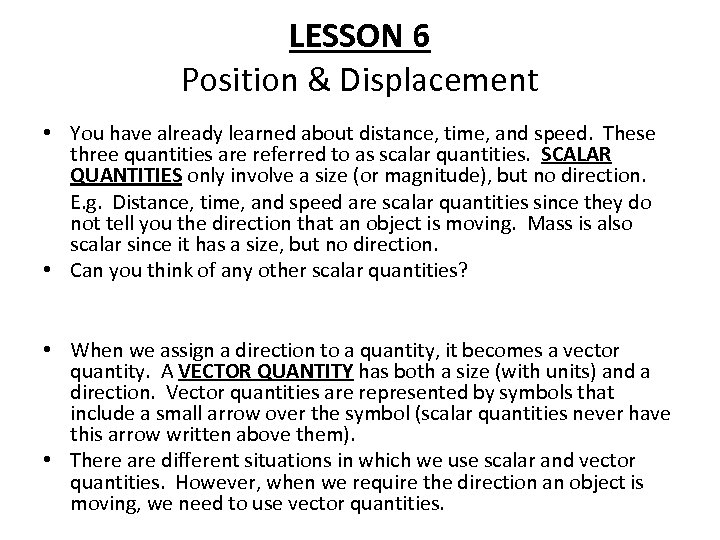LESSON 6 Position & Displacement • You have already learned about distance, time, and speed. These three quantities are referred to as scalar quantities. SCALAR QUANTITIES only involve a size (or magnitude), but no direction. E. g. Distance, time, and speed are scalar quantities since they do not tell you the direction that an object is moving. Mass is also scalar since it has a size, but no direction. • Can you think of any other scalar quantities? • When we assign a direction to a quantity, it becomes a vector quantity. A VECTOR QUANTITY has both a size (with units) and a direction. Vector quantities are represented by symbols that include a small arrow over the symbol (scalar quantities never have this arrow written above them). • There are different situations in which we use scalar and vector quantities. However, when we require the direction an object is moving, we need to use vector quantities.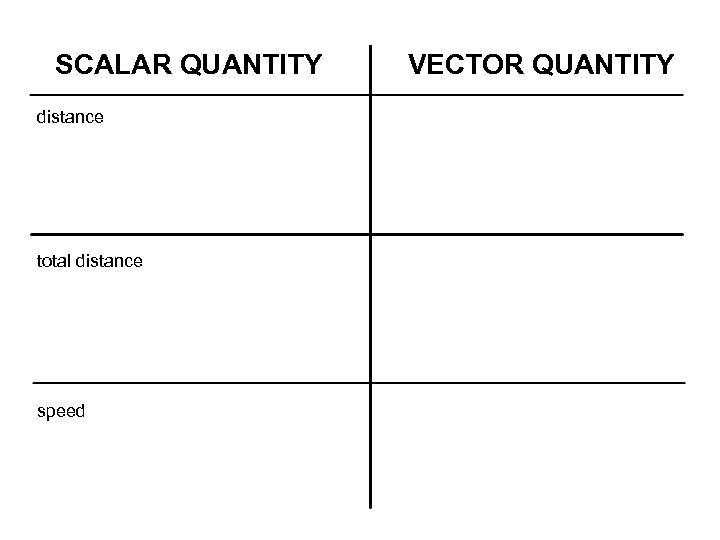SCALAR QUANTITY distance total distance speed VECTOR QUANTITY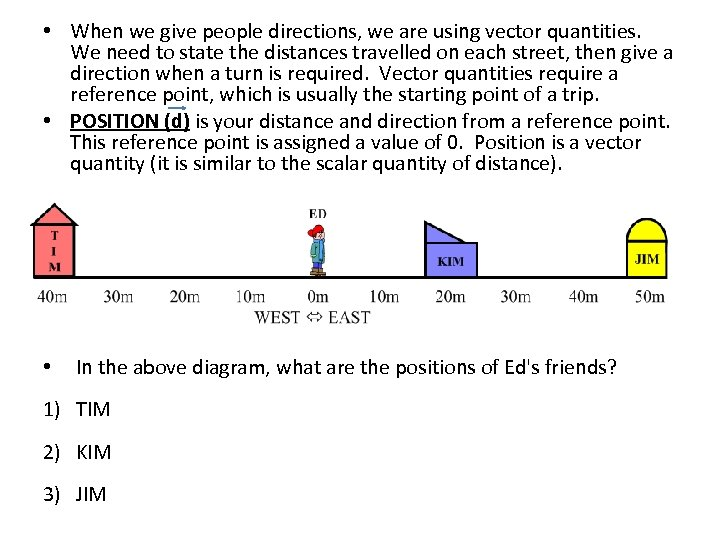• When we give people directions, we are using vector quantities. We need to state the distances travelled on each street, then give a direction when a turn is required. Vector quantities require a reference point, which is usually the starting point of a trip. • POSITION (d) is your distance and direction from a reference point. This reference point is assigned a value of 0. Position is a vector quantity (it is similar to the scalar quantity of distance). • In the above diagram, what are the positions of Ed's friends? 1) TIM 2) KIM 3) JIM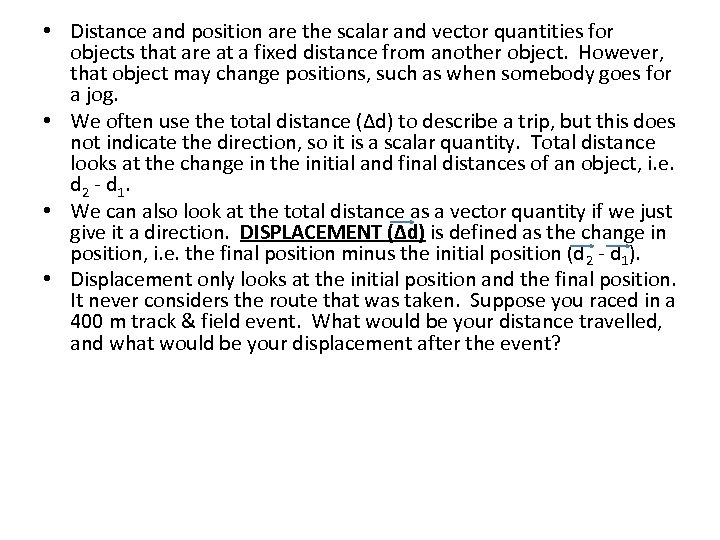• Distance and position are the scalar and vector quantities for objects that are at a fixed distance from another object. However, that object may change positions, such as when somebody goes for a jog. • We often use the total distance (Δd) to describe a trip, but this does not indicate the direction, so it is a scalar quantity. Total distance looks at the change in the initial and final distances of an object, i. e. d 2 - d 1. • We can also look at the total distance as a vector quantity if we just give it a direction. DISPLACEMENT (Δd) is defined as the change in position, i. e. the final position minus the initial position (d 2 - d 1). • Displacement only looks at the initial position and the final position. It never considers the route that was taken. Suppose you raced in a 400 m track & field event. What would be your distance travelled, and what would be your displacement after the event?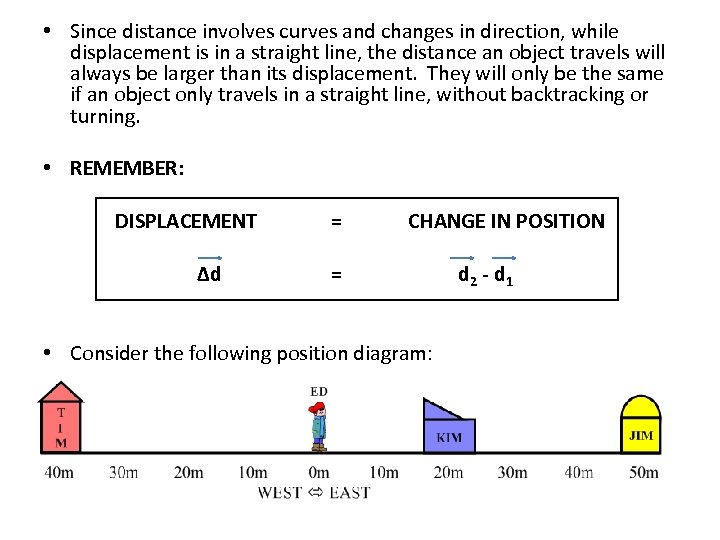• Since distance involves curves and changes in direction, while displacement is in a straight line, the distance an object travels will always be larger than its displacement. They will only be the same if an object only travels in a straight line, without backtracking or turning. • REMEMBER: DISPLACEMENT Δd = CHANGE IN POSITION = • Consider the following position diagram: d 2 - d 1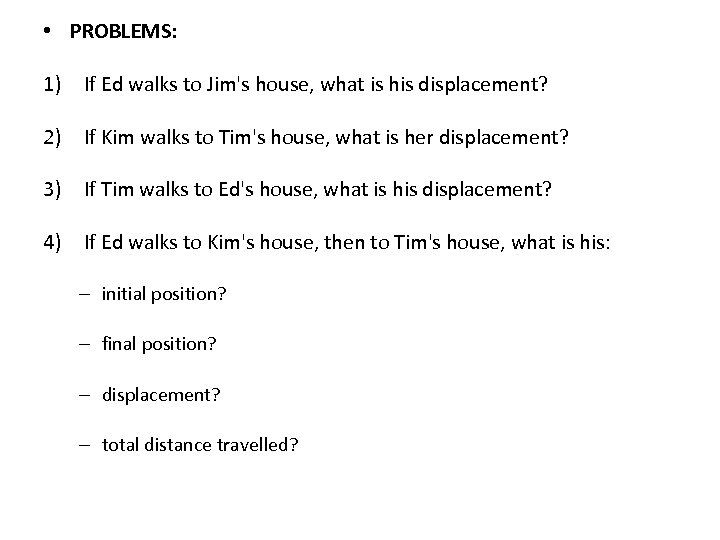• PROBLEMS: 1) If Ed walks to Jim's house, what is his displacement? 2) If Kim walks to Tim's house, what is her displacement? 3) If Tim walks to Ed's house, what is his displacement? 4) If Ed walks to Kim's house, then to Tim's house, what is his: – initial position? – final position? – displacement? – total distance travelled?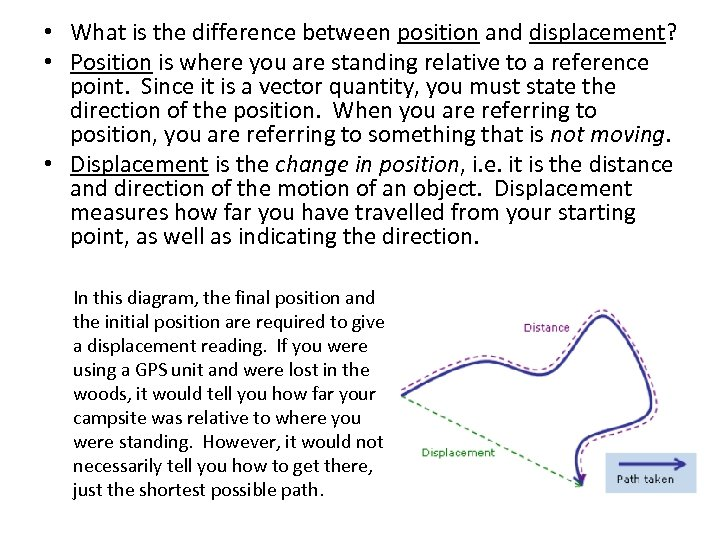• What is the difference between position and displacement? • Position is where you are standing relative to a reference point. Since it is a vector quantity, you must state the direction of the position. When you are referring to position, you are referring to something that is not moving. • Displacement is the change in position, i. e. it is the distance and direction of the motion of an object. Displacement measures how far you have travelled from your starting point, as well as indicating the direction. In this diagram, the final position and the initial position are required to give a displacement reading. If you were using a GPS unit and were lost in the woods, it would tell you how far your campsite was relative to where you were standing. However, it would not necessarily tell you how to get there, just the shortest possible path.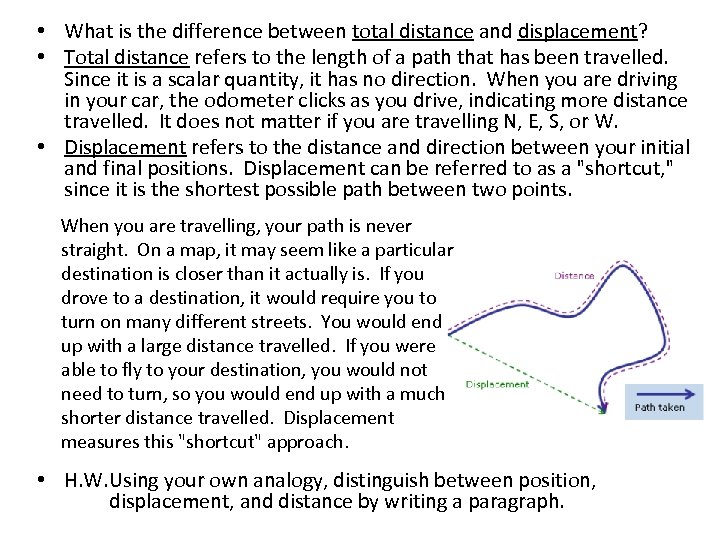• What is the difference between total distance and displacement? • Total distance refers to the length of a path that has been travelled. Since it is a scalar quantity, it has no direction. When you are driving in your car, the odometer clicks as you drive, indicating more distance travelled. It does not matter if you are travelling N, E, S, or W. • Displacement refers to the distance and direction between your initial and final positions. Displacement can be referred to as a "shortcut, " since it is the shortest possible path between two points. When you are travelling, your path is never straight. On a map, it may seem like a particular destination is closer than it actually is. If you drove to a destination, it would require you to turn on many different streets. You would end up with a large distance travelled. If you were able to fly to your destination, you would not need to turn, so you would end up with a much shorter distance travelled. Displacement measures this "shortcut" approach. • H. W. Using your own analogy, distinguish between position, displacement, and distance by writing a paragraph.